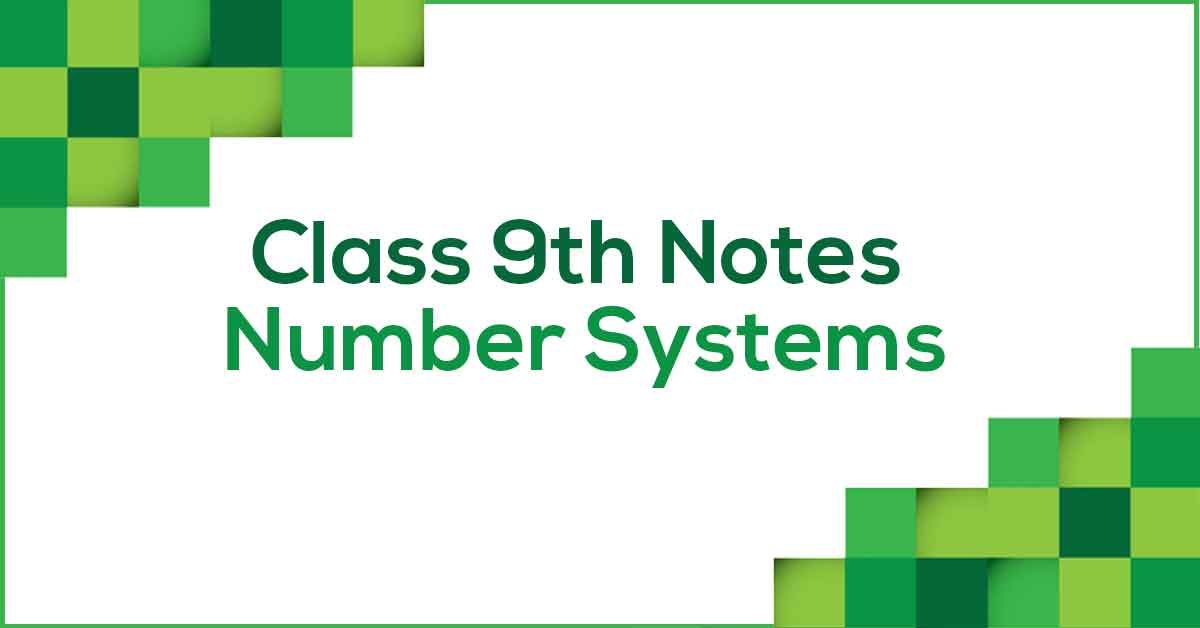Number Systems Class 9 Notes | Vidyakul
×

# Number Systems Class 9 Notes## Class 9 Maths Chapter 1 Number Systems Notes - PDF Download

Number System Class 9 Notes are prepared according to the latest NCERT Syllabus and provide the students with the summary of the chapter, important points to remember and detailed explanation of important concepts and derivations for better understanding and retaining of the chapter. Going through these Number System Class Notes for CBSE would ease out your learning and revision process.

### What do you understand by Number System?

Stated simply, a number system is a set of objects (often numbers), operations, and the rules governing those operations. One example is our familiar real number system, which uses base ten numbers and such operations as addition and multiplication. Another example is the binary number system, which uses binary addition and multiplication.